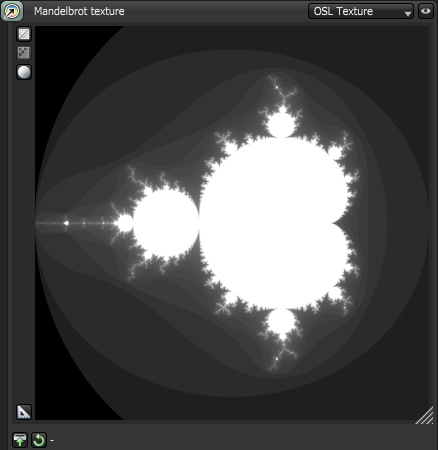# The Octane OSL guide¶

This guide describes working with OSL shaders in Octane. OSL shaders allow for custom procedural textures or custom texture mappings.

Octane supports 3 types of shaders:

• Texture: this is a shader texture which outputs a color, and can be used for material input channels and a few other places like environment textures.

• Projection: similar to a texture shader, but outputs a point-like variable. This shader can be connected to the projection input of a texture, and allows you to implement custom texture projections.

• Camera: implements a custom camera type. See camera shaders

An OSL shader can define a number of inputs, and it can contain additional information to format these inputs in user interfaces.

  1 2 3 4 5 6 7 8 9 10 11 12 13 14 15 16 17 18 19 20 21 22 23 24 25 26 27 28 29 30 31 32 33 34 35 36 37 38 39 40 41 42 43 44 45 46 47 48 49 50 51 52 53 shader Mandelbrot( output color c = 0, int maxcount = 10 [[int min=1, int max=20000, int sliderexponent=4, string label = "Max iterations", string description = "Maximal amount of iterations (z = z² + c)"]], int outputScale = 10000 [[int min=1, int max=20000, int sliderexponent=4, string label = "Iterations scale factor", string description = "Scale factor, iteration count mapped to almost white"]], float gamma = 1.0 [[string label = "Gamma", float min=0.1, float max=10]], point proj = point(u, v, 0) [[string label = "Projection"]], matrix xform = 1 [[string label = "UV transform"]] ) { // safety check: max count should not be arbitrarily high: int maxcount2 = min(maxcount, 20000); // get a point. This implements the usual UV transform + projection inputs // of Octane texture nodes point p = transform(1/xform, proj); float cx = p; float cy = p; // Iterations float x = cx; float y = cy; int count = 0; while (x*x + y*y < 4 && count < maxcount2) { count += 1; // z = z² + c float x2 = x*x - y*y + cx; y = 2*x*y + cy; x = x2; } // output value: 1.0 if the iteration doesn't diverge, < 1.0 otherwise if (count < maxcount) { float h = (float)count; c = pow(h / outputScale, gamma); c = min(c, .99); } else { c = 1.0; } } 

After compiling this texture can be rendered like any other Octane texture:The OSL standard is available from Sony Pictures Imageworks via GitHub. The OSL implementation in Octane is based on version 1.11.

Warning

When using OSL, you should ensure shaders never lock up or run for exceedingly long times. This may cause the system to freeze, or to reset the display driver.

Warning

Some operations, like out-of-bounds array access, may cause kernel crashes.

Last update: June 8, 2022